Journal of Vibroengineering

Published: 21 October 2022

# Bearing fault diagnosis method based on Gramian angular field and ensemble deep learning

Yanfang Han1
Baozhu Li2
Yingkun Huang3
Liang Li4
1Sichuan College of Architectural Technology, Chengdu, 610399, China
2Internet of Things and Smart City Innovation Platform, Zhuhai Fudan Innovation Institute, Zhuhai, 518057, China
3High Performance Computing Department, National Supercomputing Center in Shenzhen, Shenzhen, 518055, China
4College of Electrical Engineering, Southwest Jiaotong University, Chengdu, 610031, China
Corresponding Author:
Baozhu Li
Views 118

#### Abstract

Inspired by the successful experience of convolutional neural networks (CNN) in image classification, encoding vibration signals to images and then using deep learning for image analysis to obtain better performance in bearing fault diagnosis has become a highly promising approach. Based on this, we propose a novel approach to identify bearing faults in this study, which includes image-interpreted signals and integrating machine learning. In our method, each vibration signal is first encoded into two Gramian angular fields (GAF) matrices. Next, the encoded results are used to train a CNN to obtain the initial decision results. Finally, we introduce the random forest regression method to learn the distribution of the initial decision results to make the final decisions for bearing faults. To verify the effectiveness of the proposed method, we designed two case analyses using Case Western Reserve University (CWRU) bearing data. One is to verify the effectiveness of mapping the vibration signal to the GAFs, and the other is to demonstrate that integrated deep learning can improve the performance of bearing fault detection. The experimental results show that our method can effectively identify different faults and significantly outperform the comparative approach.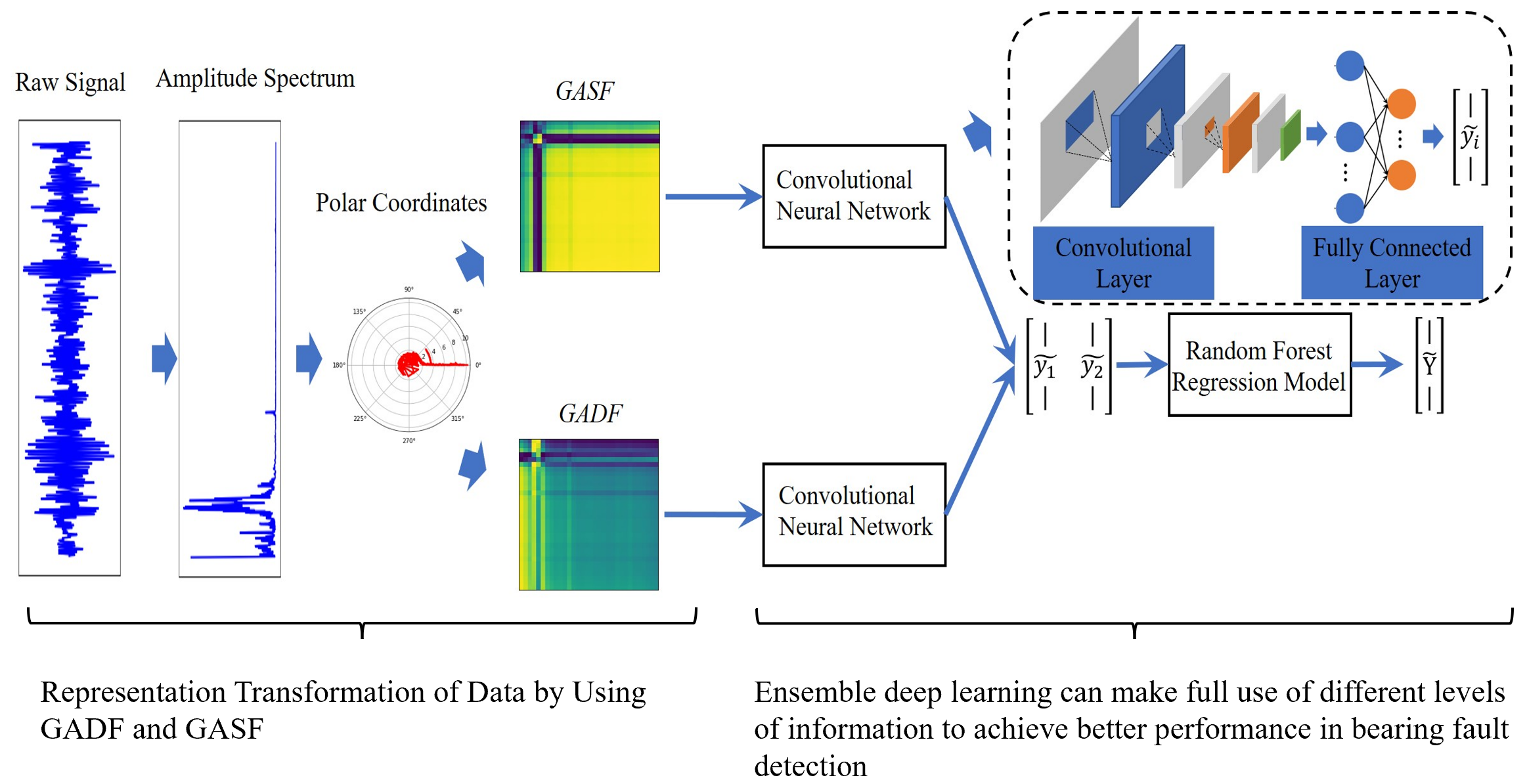#### Highlights

• In this study we model a new bearing fault detection method.
• The method is composed of data preprocessing, image-interpreted vibration signal, and ensemble deep learning.
• We use GAFs approach to construct the representation of data because GAFs contain temporal correlation of vibration signal.
• We construct an integrated deep model to achieve a high accuracy rate of bearing fault detection.
• We conclude that our method can obtain a better performance of bearing fault detection on CWRU dataset.

## 1. Introduction

In modern industries, machine health monitoring is a prerequisite for maintaining the proper operation of industrial machines. Breakdowns in industrial machines can cause huge financial losses and even pose a threat to the people who use them. Therefore, the need for better and smarter machine health-monitoring technologies has never ceased . Rolling bearings are considered the most common and critical mechanical components in rotating machinery, and their health can have a significant impact on the performance, stability, and service life of the machine. Because rolling bearings are usually in harsh operating environments, they are prone to failure during operation. Failure to detect defects in time can lead to unplanned machine downtime or even catastrophic damage. Therefore, rolling bearing fault detection is essential for the safe and reliable operation of machinery and production .

Recently, several bearing fault recognition methods have been proposed. Learning-based (including statistical learning methods and neural network methods) recognition methods can capture mechanical fault information by learning historical data and thereby enabling the automated analysis of bearing faults. The flow chart of these methods usually includes data preprocessing, feature extraction, and classifier design. Although a well-designed classification algorithm is a prerequisite for automated bearing fault detection , data preprocessing and feature extraction are also important steps.

Designing manual features based on the signal mechanism is a hot field for bearing fault diagnosis. Chen et al.  merged the bearing signal features of the time and frequency domains and then inputted these features into a deep fully connected network for fault detection. Bao et al.  calculated the L-kurtosis feature in the envelope spectrum of a vibration signal to detect pulse periodicity. Chen et al.  first transformed a vibration signal into the spectrum domain and extracted the mapping amplitude entropy as a learnable feature. Zhao et al.  first performed empirical mode decomposition (EMD) on a vibration signal, and then selected the top few-mode components containing the main information of the signal to extract the sample entropy. Liu et al.  proposed a feature extraction method based on variational mode decomposition and singular value decomposition (SVD). Unfortunately, these manual feature extraction processes are laborious and unfriendly to machine-learning designers.

Fig. 1The flow chart of the proposed method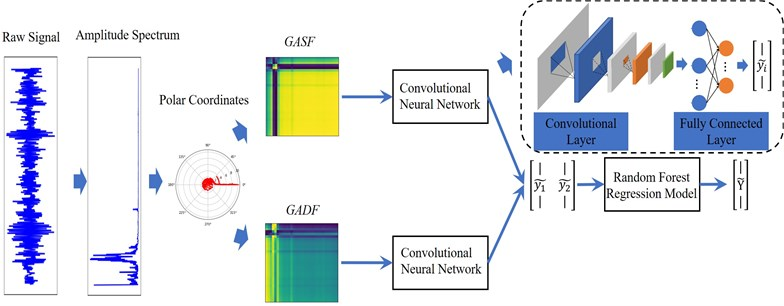Owing to the powerful feature learning ability of deep learning techniques, many researchers have attempted to introduce deep learning into the field of fault detection. Example include convolutional neural network (CNN)-based methods , , , -, sparse auto-encoder (SAE)-based methods , , and recursive neural network (RNN)-based methods , , etc. Because the original signal is easily affected by noise, the signal is often transformed into an amplitude-frequency domain sequence. Generally speaking, CNN models are good at learning deep features from image data; thus, one-dimensional vibration signals encoded as two-dimensional image data have attracted much attention. CNN and their improved models have been successfully applied to image classification because they can extract robust features directly from two-dimensional images. Many image-interpreting vibration signal approaches have been proposed. Ding et al.  proposed a method for reconstructing a two-dimensional wavelet packet energy image (WPI) of the frequency space. The WPI can represent the dynamic structure of the wavelet packet energy distribution of different bearing faults. However, the WPI method combines the wavelet packet transform and phase space reconstruction technique, which not only has high time complexity but also loses the information of the original signal when performing multiple transformations of the representation space. Mantas et al.  converted the time series to the Permutation Entropy (PE) pattern, which is a 2D digital image with multiscale time delays. However, the method ignores the amplitude information of the time series, and it is sensitive to noise in the view of the principle behind the method. Wang et al.  combined Symmetrized Dot Pattern (SDP) with CNN for intelligent bearing fault diagnosis. SDP method  converts the time series into polar SDP images, which have the frequency and amplitude of the raw signals. In  and , they convert the time series into a 2D gray image. In addition, a variety of methods use the time-frequency image to represent the time series , , but the time-frequency analysis methods cost a lot of time so it is difficult for actual online diagnosis. Wang et al.  encoded a time series as the GAF image and then took advantage of the deep model for image representation learning to obtain a better classification accuracy rate. The algorithm was validated on a 20-time series dataset and outperformed the traditional k-NN+DTW method, which was earlier considered the most effective method for time series classification.

The advantages of image-interpreting time series as a GAF image include the following: 1) there is no spatial transformation of the original time series, and the encoding process is performed in the original representation space. In other words, the time complexity of the algorithm is of the linear order Ο($n$), where n is the length of the time series. 2) The principle of the approach is simple, easy to understand, and reproducible. Surprisingly, the GAF pictorial method has rarely been applied to bearing fault detection problems. The GAF pictorial method has two different representations, including the Gramian Angular Summation Field (GASF) and the Differential Difference Field (GADF). The GASF and GADF provide different levels of information, such that, the final decision results of using them for deep learning are different. Given this, this study introduces the stacking generalization method ,  to fuse the initial decision results based on GASF and GADF. The proposed method improved the classification accuracy rate of bearing faults and increased the reliability of the results.

The flow of the proposed approach is shown in Fig. 1. It can be seen that the method is composed of data preprocessing, image-interpreted vibration signal, and ensemble deep learning. The image-interpreting stage was used to better explore the vibration signals information. Ensemble deep learning can make full use of different levels of information to achieve better performance in bearing fault detection.

In sum, the main contributions of this study are as follows:

1) The GAFs approach is introduced to encode the bearing vibration signal into GASF and GADF matrices. The GAFs contain temporal correlation of vibration signal. The 2D-CNN is used to learn the deep features of the images, and then the idea of the stacking ensemble method is combined to construct an integrated deep model to achieve a high accuracy rate of bearing fault detection. It is a decision-level fusion strategy. Due to the deep learning with GASF and GADF obtains different accuracy rates, namely, they contain different information for classification. Therefore, building an ensemble model is an ideal fault detection scheme.

2) Last but not least, we design two experiments on CWRU datasets to evaluate the performance of bearing fault classification. With those comparable results, we demonstrate that our method achieves a better performance than the comparative method.

3) The rest of the paper is organized as follows: In Section 2, we introduce the principle of encoding time series as GAF images; we present the proposed method in Section 3; next, we conduct a performance test on the CWRU dataset. Finally, in Section 5, we conclude the paper.

## 2. Image-interpreted time series

Encoding vibration signals into images of different granularities is a popular research area for bearing fault diagnosis. Here, we introduce the GAF encoding method, which first transforms the vibrating bearing signal into a 2D image, and then uses a 2D-CNN to learn the knowledge of the image.

### 2.1. GAF encoding method

Given a time series $X=\left\{{x}_{1},{x}_{2},\cdots ,{x}_{n}\right\}$, where ${x}_{i}$ is scaled in $\left[-1,1\right]$ or $\left[0,1\right]$ using:

1
$\stackrel{~}{{x}_{i}}=\frac{{x}_{i}-max\left(X\right)+{x}_{i}-min\left(X\right)}{max\left(X\right)-min\left(X\right)},$
2
$\stackrel{~}{{x}_{i}}=\frac{{x}_{i}-min\left(X\right)}{max\left(X\right)-min\left(X\right)}.$

Then, the $\stackrel{~}{X}$ can be encoded as the angular cosine and the timestamp as the radius using Eq. (3):

3

where ${t}_{i}$ is the timestamp and $N$ is a constant factor. Based on this, a one-dimensional time series is mapped to the two-dimensional image. Because ${x}_{i}\in \left[-1,1\right]$ or ${x}_{i}\in \left[0,1\right]$, $\mathrm{c}\mathrm{o}\mathrm{s}\left(\varphi \right)$ ($\varphi \in \left[0,\pi \right]$) is monotonic, such that GAF encoding is bijective. In other words, a time series can be mapped only to a unique polar coordinate space. In addition, the time dependence is preserved by the $r$ coordinates.

Fig. 2GASF images of 10 bearing status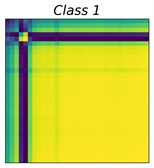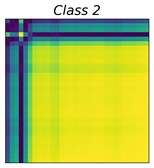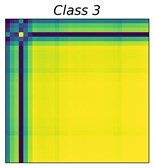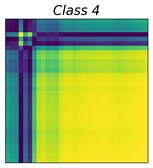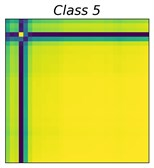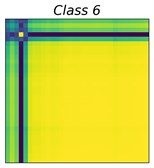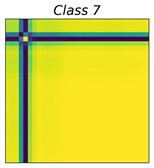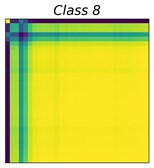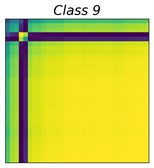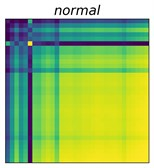Fig. 3GADF images of 10 bearing status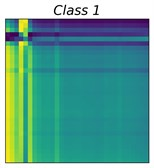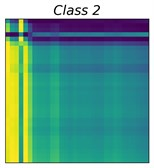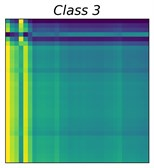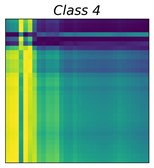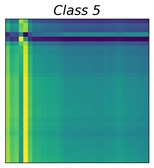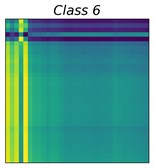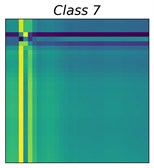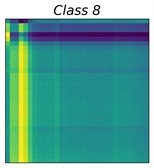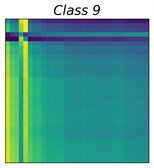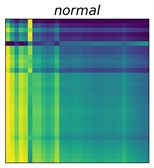Once performing the GAF encoding for time series, the temporal correlations within different time intervals are identified by considering the triangular sum/difference between each point:

4
$GASF\left(i,j\right)=\mathrm{c}\mathrm{o}\mathrm{s}\left({\varphi }_{i}+{\varphi }_{j}\right)=\stackrel{~}{{x}_{i}}\stackrel{~}{{x}_{j}}-\sqrt{1-{\left(\stackrel{~}{{x}_{i}}\right)}^{2}}\sqrt{1-{\left(\stackrel{~}{{x}_{j}}\right)}^{2}},$
5
$GADF\left(i,j\right)=\mathrm{s}\mathrm{i}\mathrm{n}\left({\varphi }_{i}-{\varphi }_{j}\right)=\stackrel{~}{{x}_{j}}\sqrt{1-{\left(\stackrel{~}{{x}_{i}}\right)}^{2}}-\stackrel{~}{{x}_{i}}\sqrt{1-{\left(\stackrel{~}{{x}_{j}}\right)}^{2}}.$

The GAF matrix was constructed in the original representation space of the time series. Therefore, the encoding method has significant advantages in terms of efficiency, while avoiding temporal information loss in the process of representation space transformation . Work  pointed out that the GAF has two significant advantages. First, the GAF matrix preserves the time dependence of the time series, that is, each element of the GAF matrix was generated sequentially from the top left to the bottom right according to the temporal order of the original time series. Second, the GAF matrix contains temporal correlations; for example, $GAF\left(i,j\right)$ denotes the time interval $k=\left|i-j\right|$ relative correlation in the direction.

In practical applications, direct encoding of the bearing vibration signal in the time domain into a GAF matrix is often unsatisfactory. This is because the bearing signal in the time domain can easily be contaminated by noise. Therefore, we encoded the amplitude-spectrum sequence of the bearing signal into the GAF image. In the amplitude spectrum, the energy of useful information is concentrated in a narrow range of frequency bands, whereas the noise energy is distributed over the entire frequency band. Assuming that $\stackrel{-}{X}=\left\{\stackrel{-}{{x}_{1}},\stackrel{-}{{x}_{2}},\cdots ,\stackrel{-}{{x}_{n}}\right\}$ is the amplitude spectrum of a bearing vibration signal, such that $\stackrel{-}{X}$ can be denoised according to Eq. (6):

6

where, $\mu$ is the mean value of $X$. As the amplitude spectrum of the vibration signal is symmetric, it is possible to consider only the left amplitude spectrum. Fig. 2 and 3 show the GASF and GADF images of 10 different bearing faults, respectively. The class distributions of these faults are shown in Table 1.

Table 1Class distribution of 9 types of faults

 Fault type Load (HP) Speed (rpm) Ball Inner race Outer race 0.007" 0 1797 class 1 class 4 class 7 0.014" 0 1797 class 2 class 5 class 8 0.021" 0 1797 class 3 class 6 class 9

## 3. Denoising method

In real applications, the sampling points of the time series are usually very large; therefore, it is necessary to reduce the dimensionality of the time series before GAF encoding. Considering that the piecewise aggregate approximation (PAA) algorithm  can not only preserve the basic trend of the time series but also has low time complexity, we use the PAA algorithm to pre-process the bearing vibration signal.

PAA is a simple and effective time series smoothing algorithm that preserves the trend of the time series. The time complexity of PAA is low; therefore, the PAA algorithm is widely used in time series analysis problems. Considering the time series $X=\left\{{x}_{1},{x}_{2},\cdots ,{x}_{n}\right\}$ is mapped to a new time series $\stackrel{^}{X}=\left\{\stackrel{^}{{x}_{1}},\stackrel{^}{{x}_{2}},\cdots ,\stackrel{^}{{x}_{m}}\right\}$, can be calculated using the following formula:

7

From Eq. (7), we find that $X$ is sequentially divided into $m$ blocks of equal size, and the mean value of each block is used to re-represent the block. The PAA algorithm has a certain noise reduction effect, as it uses the mean value to smooth the data. Clearly, the selection of $m$ is crucial. If $m$ is too large, the smoothed result loses the original structural information, where $m$ is too small, and the effect is not suitable for noise reduction. From Eq. (7), we can also see that the traditional PAA needs to satisfy $n/m$ as an integer. For $n/m$ is a non-integer number that can be found in  and .

### 3.1. Stacking integration methodology

Bagging, boosting, and stacking methods are three commonly used ensemble learning methods. The bagging method is an algorithm to reduce the variance in the estimate by using voting or mean reversion to achieve the fusion of multiple decision results . Boosting method can upgrade weak learners to strong learners. Unlike the parallel learning approach of the bagging method, boosting method is a sequential framework. Boosting method works by sequentially training an initial learner from the training set, and then adjusting the distribution of training samples according to the results of the initial learner, thus, those instances of wrong decisions of the previous initial learner will be received attention. AdaBoost  method is a very classic boosting method. The stacking method is a different fusion method that is essentially representation learning. The principle of the stacking method is that they perform the second-stage learning with the result of the initial learner. Stacking method has yielded unusually brilliant results in many data mining competitions (e.g., data science competitions on the Kaggle platform). For example, in the solution proposed by the grand prize winner of the 2009 Netflix recommendation competition, integrating multiple initial learners is the core of its design .

In the case of the classification task, the basic process of the stacking method is to learn different classification algorithms ${\mathcal{L}}_{1},\cdots ,{\mathcal{L}}_{K}$ on the dataset $D$. ${d}_{i}=\left({x}_{i},{y}_{i}\right)\in D$ is an instance, where ${x}_{i}$ is the feature vector and ${y}_{i}$ is the corresponding label. In the first stage of the stacking method, a set of base classifiers ${C}_{1},\cdots ,{C}_{K}$ where are generated. In the second stage, a meta-classifier was learned based on the outputs of the base classifiers. Note that the leave-one-out method or cross-validation  was applied to generate the training set for learning the meta-classifiers . For the leave-one-out method, each base classifier uses almost all examples and leaves the remaining one for testing. The procedure can be formalized as $\forall i=1,\cdots ,n$ ($n$ is the number of examples), ${C}_{t}^{i}={\mathcal{L}}_{t}\left(D-{d}_{i}\right)$, $\forall t=1,\cdots ,K$, and next, the base learner is used to classify ${d}_{i}$ by ${\stackrel{~}{y}}_{i}^{t}={C}_{t}\left({x}_{i}\right)$. Therefore, ${d}_{i}$ can be reconstructed to a new vector $\left(\left({\stackrel{~}{y}}_{i}^{1},\cdots ,{\stackrel{~}{y}}_{i}^{K}\right),{y}_{i}\right)$. The inputs of the meta-learning phase comprised the predictions of the base classifier.

As the leave-one-out method reconstructs only a sample per learning, it increases the time cost of the reconstruction step, whereas cross-validation predicts a pre-defined subset of the original sample set at a time and gets the predictions of the base classifier on these subsets. Thus, cross-validation is preferred for applying the stacking algorithm for big data.

## 4. Experiments

Here, we used CWRU bearing data  to verify the effectiveness of the proposed method. The dataset comprised a multivariate vibration time series generated by the bearing test equipment, as shown in Fig. 4. In this study, the bearing dataset included the following four conditions: normal, outer race failure, inner race failure, and roller failure. The drive-side vibration signal was used with a sampling rate of 48 kHz and a motor load of 0 hp. Table 1 shows the class distributions of the selected data.

Fig. 4Test-stand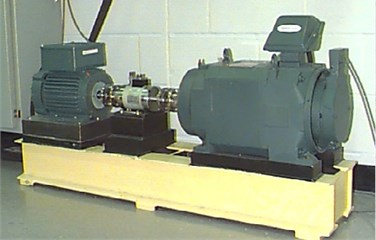Table 2Accuracy rate of 5 methods on raw CWRU

 Algorithm GASF+2D-CNN GADF+2D-CNN WPI+2D-CNN Amplitude spectrum + 1D-CNN Ensemble method acc 99.4 % 99.8 % 98.7 % 75.6 % 100 %

## 5. Experimental design

Here, we used a 2D-CNN as the initial learner. Considering useable data is small, the 2D-CNN model includes only three convolutional layers, three pooling layers, and one fully connected structure. Meanwhile, the batch normalization  and the dropout  methods are used to reduce the risk of deep model over-fitting. The 2D-CNN uses the cross-entropy loss function, and Adam optimizes the algorithm . We used the random forest regression method as the meta-learner. We follow the common practice of dividing the CWRU dataset was divided into the training set, test set, and validation set in the ratio of 0.5, 0.3, and 0.2. The training set was used to train the 2D-CNN and the validation set was used to train the random regression model. The experiments are repeated 10 times under different random seeds, and the final results are taken as the mean value of the 10 experiments. Considering the uniform distribution of classes, performing different algorithms can be well measured by the traditional classification accurate rate:

8

### 5.1. Raw CWRU data

In this section, the experiments were divided into two parts. The first was to verify the validity of the image interpretation of vibration signals. Its purpose was to compare the fault recognition performance before and after image encoding. We used the 1D-CNN model to learn one-dimensional vibration signals and applied the 2D-CNN model to the imaged data. Second, we compare the performance of the proposed method with that of the existing method in  (hereafter referred to as WPI+2D-CNN). The topological structure of 2D-CNN is described in the above section. In addition, the 1D-CNN model contains a one-dimensional convolutional layer, a pooling layer, and a fully connected structure. To reduce the overfitting of CNN, batch normalization and dropout are used to reduce the risk of overfitting.

We showed the accuracy rates on the original CWRU data in Table2. From the table, we can conclude that: 1) the vibration signal encoded to a two-dimensional image can be better learned to achieve better performance; 2) the proposed ensemble method gets better performance than the existing method. Although WPI+2D-CNN also gets the accurate rate close to our approach, it has a high time cost than the proposed method. Due to GAFs performing the outer product of the time series, so the time complexity of GAFs is $\mathrm{O}\left({N}^{2}\right)$, $N$ is the length of time series. Work  does not give the time complexity of WPI, such that we add a test to compare their runtimes. Table 3 shows the time consumption of imaging the raw CWRU data using GAFs and WPI respectively. From the table, we can see that WPI costs 2938.267 seconds, which is awful larger than GASF and GADF.

Table 3Runtime of GAFs and WPI

 GASF GADF WPI Runtime (s) 2.289 (s) 2.236 (s) 2938.267 (s)

Why can fusing the knowledge of both GASF and GADF can improve accurate rates? From Fig. 2, we can see that class 5, class 6, and class 7 have a similar representation in the GASF image while presenting a significant difference in the GADF domain. In the same light, class 2 and class 3 have similar GADF features, instead, have different GASF features. Fig. 2 and 3 explain the advantages of our method. In fact, from the perspective of information theory, fusing different representation features of the GAF can increase the information entropy of the inputs and help improve the accuracy of the learning-based prediction model.

To further verify the robustness of our method, we added Gaussian white noise to raw CWRU data. We added noise to the vibration signal with SNR of [–5 dB, –2 dB, 2 dB, 5 dB]. The experimental results are in Table 4. From the table, we can see that: 1) the proposed method can achieve better performance than WPI+2D-CNN in a noisy environment. 2) The fault identification performance of GADF+2D-CNN is close to GASF+2D-CNN. 3) The ensemble model fusing the GAF image information can achieve excellent performance. Note that for the bearing signal with complex noise, we can choose advanced denoising methods instead of formula (6). Examples include deep learning, wavelet shrinkage-based, SVD-based methods, and EMD-based methods.

In summary, we can conclude that fusing the knowledge of the GASF and the GADF can improve the performance of bearing fault diagnosis for the CWRU dataset. Considering that the time cost of building the GAF was low, the proposed method was more in line with the practical application requirements.

Table 4Accuracy rate of 5 methods on noisy CWRU

 –5 dB –2 dB 2 dB 5 dB WPI+2D-CNN 87.1 % 93.2 % 97.6 % 97.8 % GASF+2D-CNN 99.0 % 99.0 % 99.1 % 99.4 % GADF+2D-CNN 99. 4 % 99.1 % 99.0 % 99.6 % Ensemble method 99.8 % 100 % 100 % 100 %

## 6. Conclusions

This study proposed a bearing fault detection method that combines im-age-interpreting vibration signals and integrating deep learning, which can realize the accurate identification of bearing faults. Our method encoded one-dimensional vibration signals into a two-dimensional image and then used a 2D-CNN to obtain the initial decision result. Finally, we introduced a decision layer integration method to realize the fusion of multiple underlying decisions. Experiments on the CWRU real-world dataset show that the proposed method can obtain a better recognition accuracy rate than the existing method (i.e., WPI+2D-CNN), even when Gaussian white noise is added to the original vibration signal.

Altogether, the learning-based method for bearing fault detection is provided in this work. Next, we plan to apply our method to different publicly available bearing failure datasets and laboratory datasets.

#### Acknowledgements

This work is supported by the National Natural Science Foundation of China (Grant No. 61901191), and the Shandong Provincial Natural Science Foundation (Grant No. ZR2020LZH005).